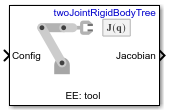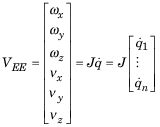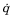Get Jacobian

Geometric Jacobian for robot configuration

• Library:
• Robotics System Toolbox / Manipulator Algorithms

•Description

The Get Jacobian block returns the geometric Jacobian relative to the base for the specified end effector at the given configuration of a rigidBodyTree robot model.

The Jacobian maps the joint-space velocity to the end-effector velocity relative to the base coordinate frame. The end-effector velocity equals:ω is the angular velocity, υ is the linear velocity, andis the joint-space velocity.

Ports

Input

expand all

Robot configuration, specified as a vector of positions for all nonfixed joints in the robot model, as set by the Rigid body tree parameter. You can also generate this vector for a complex robot using the homeConfiguration or randomConfiguration functions inside a Constant or MATLAB Function block.

Output

expand all

Geometric Jacobian of the end effector with the specified configuration, Config, returned as a 6-by-n matrix, where n is the number of degrees of freedom of the end effector. The Jacobian maps the joint-space velocity to the end-effector velocity relative to the base coordinate frame. The end-effector velocity equals:ω is the angular velocity, υ is the linear velocity, andis the joint-space velocity.

Parameters

expand all

Robot model, specified as a rigidBodyTree object. You can also import a robot model from an URDF (Unified Robot Description Formation) file using importrobot.

The default robot model, twoJointRigidBodyTree, is a robot with revolute joints and two degrees of freedom.

End effector for Jacobian, specified as a body name from the Rigid body tree robot model. To access body names from the robot model, click .

• Interpreted execution — Simulate model using the MATLAB® interpreter. This option shortens startup time but has a slower simulation speed than Code generation. In this mode, you can debug the source code of the block.

• Code generation — Simulate model using generated C code. The first time you run a simulation, Simulink® generates C code for the block. The C code is reused for subsequent simulations, as long as the model does not change. This option requires additional startup time, but the speed of the subsequent simulations is comparable to Interpreted execution.

Tunable: No

Extended Capabilities

C/C++ Code GenerationGenerate C and C++ code using Simulink® Coder™.

Introduced in R2018a